# Nesterov’s Accelerated Gradient Descent for Smooth and Strongly Convex OptimizationAbout a year ago I described Nesterov’s Accelerated Gradient Descent in the context of smooth optimization. As I mentioned previously this has been by far the most popular post on this blog. Today I have decided to revisit this post to give a slightly more geometrical proof (though unfortunately still magical in various parts). I will focus on unconstrained optimization of a smooth and strongly convex function(in the previous post I dealt only with the smooth case). Preciselyis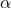-strongly convex and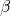-smooth, and we denote by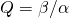the condition number of. As explained in this post, in this case the basic gradient descent algorithm requiresiterations to reach-accuracy. As we shall see below Nesterov’s Accelerated Gradient Descent attains the improved oracle complexity of. This is particularly relevant in Machine Learning applications since the strong convexity parametercan often be viewed as a regularization term, and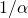can be as large as the sample size. Thus reducing the number of step from “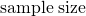” to “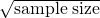” can be a huge deal, especially in large scale applications.

Without further due let’s get to the algorithm, which can be described quite succintly. Note that everything written below is simply a condensed version of the calculations appearing on pages 71–81 in Nesterov 2004’s book. Start at an arbitrary initial point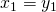and then iterate the following equations for,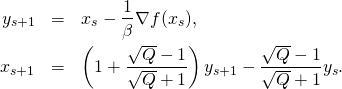Theorem Letbe-strongly convex and-smooth, then Nesterov’s Accelerated Gradient Descent satisfiesProof: We define-strongly convex quadratic functions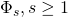by induction as follows:

(1)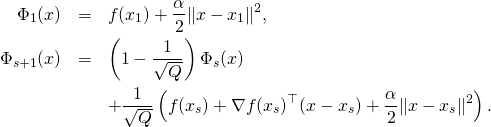Intuitivelybecomes a finer and finer approximation (from below) toin the following sense:

(2)The above inequality can be proved immediately by induction, using the fact that by-strong convexity one hasEquation (2) by itself does not say much, for it to be useful one needs to understand how “far” belowis. The following inequality answers this question:

(3)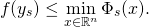The rest of the proof is devoted to showing that (3) holds true, but first let us see how to combine (2) and (3) to obtain the rate given by the theorem (we use that by-smoothness one has):We now prove (3) by induction (note that it is true atsince). Let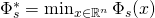. Using the definition of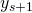(and-smoothness), convexity, and the induction hypothesis, one gets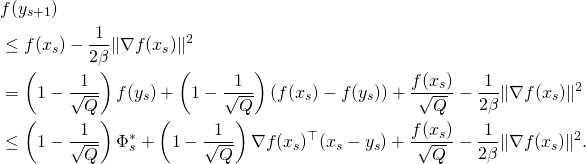Thus we now have to show that

(4)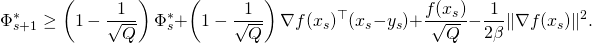To prove this inequality we have to understand better the functions. First note that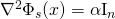(immediate by induction) and thushas to be of the following form:for some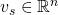. Now observe that by differentiating (1) and using the above form ofone obtains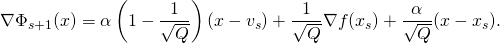In particularis by definition minimized atwhich can now be defined by induction using the above identity, precisely:

(5)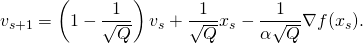Using the form ofand, as well as the original definition (1) one gets the following identity by evaluatingat:

(6)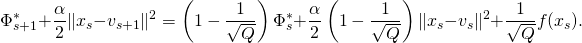Note that thanks to (5) one has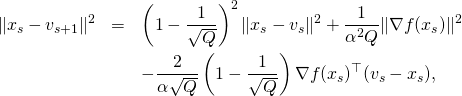which combined with (6) yieldsFinally we show by induction that, which concludes the proof of (4) and thus also concludes the proof of the theorem: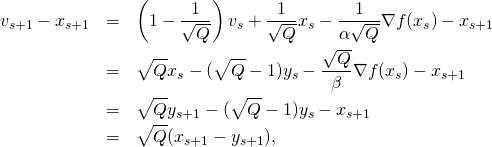where the first equality comes from (5), the second from the induction hypothesis, the third from the definition ofand the last one from the definition of.This entry was posted in Optimization. Bookmark the permalink.

## 13 Responses to "Nesterov’s Accelerated Gradient Descent for Smooth and Strongly Convex Optimization"

•••••••••••